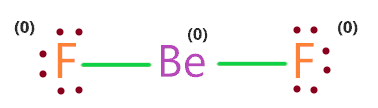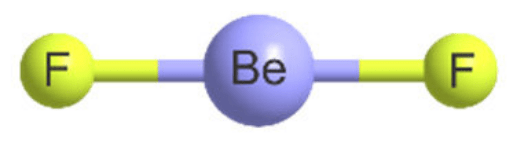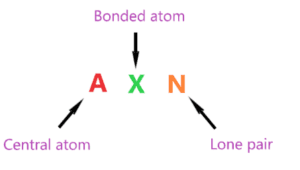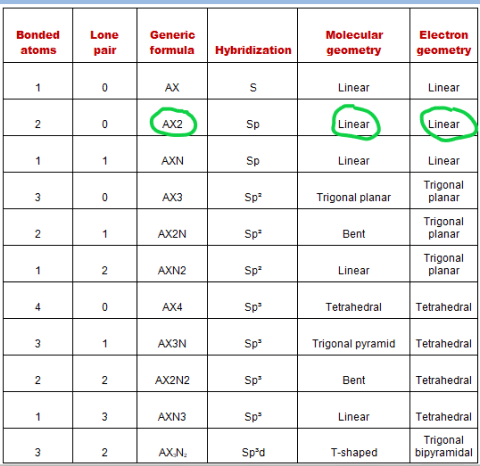# Beryllium fluoride (BeF2) lewis dot structure, molecular geometry, electron geometry, polar or nonpolar, bond angle

Home  > Chemistry Article > BeF2 lewis structure and its molecular geometryBeryllium fluoride is an inorganic compound that appears as colorless lumps have a chemical formula BeF2. It is an odorless white solid also known as fluoride salt of beryllium. It is commonly used in biochemistry.

In this tutorial, we will discuss Beryllium fluoride (BeF2) lewis dot structure, molecular geometry, electron geometry, hybridization, polar or nonpolar, its bond angle, etc.

Beryllium fluoride has distinctive optical properties and its structure resembles that of quartz. It is chemically stable and in the solid-state exist as a glass.

 Name of Molecule Beryllium fluoride Chemical formula BeF2 Molecular geometry of BeF2 Linear Electron geometry of BeF2 Linear Hybridization Sp Nature Nonpolar molecule Total Valence electron for BeF2 16
Page Contents

## How to draw lewis structure of BeF2?

BeF2 Lewis structure contains beryllium atom in central position whereas both fluorine atom surrounding to it. There is no lone pair present on the central atom but 3 lone pairs present on each outer atom in the lewis dot structure of BeF2.

BeF2 Lewis structure is exceptional to the octet rule as its central atom doesn’t require 8 electrons in the outermost shell to attains stability.

Let’s see how to draw this in a simple way.

## Follow some steps for drawing the lewis dot structure for BeF2

1. Count total valence electron in BeF2

First of all, determine the valence electron that is available for drawing the lewis structure of BeF2 because the lewis diagram is all about the representation of valence electrons on atoms.

So, an easy way to find the valence electron of atoms in the BeF2 molecule is, just to look at the periodic group of beryllium and fluorine atoms.

As beryllium atom belongs to group 2nd in periodic table and fluorine situated in 17th group, hence, the valence electron for beryllium is 2 and for fluorine atom, it is 7.

⇒ Total number of the valence electrons in beryllium = 2

⇒ Total number of the valence electrons in fluorine = 7

∴ Total number of valence electron available for the BeF2 Lewis structure = 2 + 7(2) = 16 valence electrons         [∴BeF2 molecule has one beryllium and two fluorine atom]2. Find the least electronegative atom and placed it at center

An atom with a less electronegative value is more preferable for the central position in the lewis diagram because they are more prone to share the electrons with surrounding atoms.

In the case of the BeF2 molecule, the beryllium atom is less electronegative than the fluorine atom, also, fluorine is the highest electronegative element in chemistry, hence, it always takes the surrounding position in the lewis diagram.

So, just put the beryllium in the center position and spread both fluorine atoms around it.3. Connect outer atoms to central atom with a single bond

In this step, place the single bond in between the outer atom(fluorine) and central atom(beryllium).After connecting each outer atom to the central atom, count the number of valence electrons used in the above structure. There are 2 single used in the above structure, and one single bond means 2 electrons.

Hence, in the above structure, (2 × 2) = 4 valence electrons are used from a total of 16 valence electrons available for drawing the BeF2 Lewis structure.

∴ (16 – 4) = 12 valence electrons

So, we are left with 12 valence electrons more.

4. Place remaining valence electrons starting from outer atom first

Let’s start putting the remaining valence electrons on outer atoms first (in the case of BeF2, fluorine is the outer atom) to complete the octet i.e. Each atom must contain 8 electrons to complete the octet, an exception may occur.### Lewis dot structure for BeF2

So, both fluorine atoms in the above structure completed their octet, because both of them have 8 electrons(6 represented as dots and 2 electrons in a single bond) in their outermost shell. Also, the central atom(beryllium) also completed its octet because it has 4 electrons(2 single bonds) in its outermost shell.

Beryllium atom only needs 4 valence electrons in its outermost shell to complete the octet. (Exception to the octet rule).

Beryllium atom is exception to octet rule just as H, He, Li, etc. that do not require 8 electrons to fulfill the octet. Their s-orbitals do not need 8 electrons to complete the shell to feel full, so they don’t follow the octet rule.

Now again count the number of valence electrons used in the above structure.

There are 6 electrons placed over each fluorine atom, hence, (2 × 6) = 12 valence electrons, and we had 12 valences electrons remaining(check-in 3rd step).

∴ (12 – 12) = 0 valence electrons

So, we successfully used all the valence electrons available that are available for drawing the lewis structure of BeF2.

Now we only need to verify the stability of the above BeF2 lewis structure with the help of the formal charge concept.

5. Check the stability with the help of a formal charge concept

The lesser the formal charge on atoms, the better is the stability of the lewis diagram.

To calculate the formal charge on an atom. Use the formula given below-

⇒ Formal charge = (valence electrons – lone pair electrons –  1/2 bonded pair electrons)

Let’s count the formal charge on the fluorine atom first, both fluorine atoms in the BeF2 Lewis structure(4th step) have the same bonded pair and lone pair, so, just count the F.C. for the one fluorine atom.

For fluorine atom:

⇒ Valence electrons of fluorine = 7

⇒ Lone pair electrons on fluorine = 6

⇒ Shared pair electrons around fluorine(1 single bond) = 2

∴ (7 – 6 – 2/2) = 0 formal charge on both fluorine atoms.

For beryllium atom

⇒ Valence electrons of beryllium = 2

⇒ Lone pair electrons on beryllium = 0

⇒ Shared pair electrons around beryllium (2 single bonds) = 4

∴ (2 – 0 – 4/2) = 0 formal charge on the beryllium central atom.So, all the atoms in the BeF2 Lewis structure have a formal charge equal to zero. Therefore, the above lewis structure of beryllium fluoride is most appropriate and stable.

## What are the electron and molecular geometry of BeF2?

The molecular geometry of BeF2 is linear because according to VSEPR theory, valence shell electrons around the fluorine and beryllium atoms are going to repel each other since the central atom doesn’t contain any lone pair, so, those two fluorine atoms are going to push far as much as they can to either side of beryllium central atom and that makes BeF2 is a linear molecule.### BeF2 molecular geometry

For keeping the repulsive force least generated by the electron pairs around the central atom, BeF2 acquires the linear molecular shape.

The electron geometry of BeF2 is also linear because there is no lone pair present on the central atom that can cause disorientation in the molecule.

Let’s find the molecular shape and electron geometry of BeF2 using the AXN method.AXN notation for BeF2 molecule:

• A denotes the central atom, so, in the BeF2 molecule, beryllium is the central atom. A = Beryllium
• X denotes the bonded atoms to the central atom, as we know, beryllium is bonded with two fluorine atoms. Therefore, X = 2
• N denotes the lone pair on the central atom, as per BeF2 lewis structure, beryllium does not have any lone pair. Hence, N = 0

So, the AXN generic formula for the BeF2 molecule becomes AX2N0 or AX2.

As per the VSEPR chart, if a molecule central atom is attached with two bonded atoms and has zero lone pair then the molecular and electron geometry of that molecule will be linear in nature.Hence, the molecular geometry for BeF2 is linear as well as its electron geometry is also linear.

## Hybridization of BeF2

Let’s find the hybridization of BeF2 through the steric number of its central atom.

“Steric number is the addition of a total number of bonded atoms around a central atom and the lone pair present on it.”

∴ Steric number of BeF2 = (Number of bonded atoms attached to beryllium + Lone pair on beryllium)

As per the lewis structure of BeF2, the Beryllium atom is bonded with two fluorine atoms and it contains no lone pair of electrons.

∴ Steric number of BeF2 = (2 + 0) = 2

 Steric number Hybridization 1 S 2 Sp 3 Sp² 4 Sp³ 5 Sp³d 6 Sp³d²

So, for a steric number of two, we get the Sp hybridization on the beryllium atom in the BeF2 molecule.

## The bond angle of BeF2

The bond angle of BeF2 is 180º as expected because the beryllium central atom attains Sp hybridized and no lone pair present on it. Therefore, no disorientation occurs around the central atom which makes it’s linear in shape that has a bond angle of 180º.## Beryllium fluoride polarity: is BeF2 polar or nonpolar?

Well, we know the polar molecule has some dipole moment because of unequal distribution of charges whereas the non-polar molecule has an equal distribution of charges that cause zero dipole moment because they cancel out each other due to the symmetrical shape of the molecule.

Is BeF2 polar or non-polar? BeF2 is a non-polar molecule because it contains two bonds(Be-F) that are arranged symmetrically to each other. Due to this, the dipole moment generated on both sides along the Be-F bond will cancel out each other making it’s a non-polar molecule.

Or you can say BeF2 is a nonpolar molecule because all of the constituent atoms are arranged linearly around the central atom that causes symmetric charge distribution leads to the net dipole moment in molecule zero.

## FAQ

### Why is the electron and molecule geometry of BeF2 is same?

As we know, the molecular geometry of BeF2 is linear and electron geometry is also linear because electron geometry predicts the shape of a molecule with the help of lone pair as well bond pair but molecular geometry has only taken bond pair to predict the shape of the molecule.

As there is no lone pair present on the central atom in the BeF2 Lewis structure. Therefore, both molecular and electrons geometry predict the shape of BeF2 with the help of bonded pair of electrons.

Hence, the electron geometry and molecular geometry of BeF2 are the same(linear).

### Why does BeF2 lewis’s structure violate the octet rule?

Generally, the octet rule said an atom is most stable when it has 8 electrons around it, more than this an atom is called a member of the hypervalent compound or known as expanded octet.

So, in the case of the BeF2 Lewis dot structure, the beryllium central atom only gets 4 electrons in its outer shell to attain stability and violate the octet.

This is because the beryllium atom has too few electrons to form an octet since it has only two valence electrons, therefore, it can only form a maximum of two bond pairs(4 electrons in the outer shell).

Properties of Beryllium fluoride

• It has a molar mass of 47.01 g/mol.
• It appears as colorless lumps.
• It has a boiling point of 1,169 °C and a melting point of 554 °C.
• It is denser than water and highly soluble in an aqueous solution.
• It has a trigonal crystal structure.

## Summary

• The total valence electron is available for drawing the BeF2 Lewis structure is 16.
• The steric number of beryllium central atom in the BeF2 molecule is 2, thus, it forms Sp hybridization.
• BeF2 is a nonpolar molecule because of symmetrical geometry that causes the uniform distribution of charge in the molecule that leads to its net dipole moment zero.
• In the BeF2 Lewis dot structure, a total of 6 lone pairs and 2 bonded pairs are present.
• The molecular geometry of BeF2 is linear and its electron geometry is also linear in nature.
##### Subscribe to Blog via Email

Join 2 other subscribers

Share it...